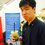# Something I realized about powers:

I was walking around (currently on vacation) and I realized something which I think is true (and I'm sure have been thought of before):

For all positive integer $b > 1$...

$\sum_{n = 0}^k b^n = \frac {b^{n+1} - 1}{b - 1}$

So I ask a few questions:

1. Can somebody prove this? I'm only a high school student so I'm not totally sure how to do this.

2. Is this true for non-integers?

3. Any relevant theorems on this?

Thanks guysNote by Michael Tong
6 years, 7 months ago

This discussion board is a place to discuss our Daily Challenges and the math and science related to those challenges. Explanations are more than just a solution — they should explain the steps and thinking strategies that you used to obtain the solution. Comments should further the discussion of math and science.

When posting on Brilliant:

• Use the emojis to react to an explanation, whether you're congratulating a job well done , or just really confused .
• Ask specific questions about the challenge or the steps in somebody's explanation. Well-posed questions can add a lot to the discussion, but posting "I don't understand!" doesn't help anyone.
• Try to contribute something new to the discussion, whether it is an extension, generalization or other idea related to the challenge.

MarkdownAppears as
*italics* or _italics_ italics
**bold** or __bold__ bold
- bulleted- list
• bulleted
• list
1. numbered2. list
1. numbered
2. list
Note: you must add a full line of space before and after lists for them to show up correctly
paragraph 1paragraph 2

paragraph 1

paragraph 2

[example link](https://brilliant.org)example link
> This is a quote
This is a quote
    # I indented these lines
# 4 spaces, and now they show
# up as a code block.

print "hello world"
# I indented these lines
# 4 spaces, and now they show
# up as a code block.

print "hello world"
MathAppears as
Remember to wrap math in $$ ... $$ or $ ... $ to ensure proper formatting.
2 \times 3 $2 \times 3$
2^{34} $2^{34}$
a_{i-1} $a_{i-1}$
\frac{2}{3} $\frac{2}{3}$
\sqrt{2} $\sqrt{2}$
\sum_{i=1}^3 $\sum_{i=1}^3$
\sin \theta $\sin \theta$
\boxed{123} $\boxed{123}$

Sort by:

Is not this, the sum of a geometric progression? I am sure you already know how to get this formula.

- 6 years, 7 months ago

Theorem: The series $\sum a_n$ converges if $\lim_{n\to \infty} \sup \sqrt[n]{}{\left |a_n \right |<1.}$

Definition:

http://en.wikipedia.org/wiki/Supremum

Let ${s_n}$ be a sequence of real numbers. Let $E$ be the set of numbers x such that $s_{n_k} \to x$ for some subsequence ${s_{n_k}}$. Put $\lim \sup x_n= \sup E.$

Proof of the Theorem:

If $\alpha=\lim_{n\to \infty} \sup \sqrt[n]{\left |a_n \right |}$ then there exists $\beta >0$ such that $\alpha< \beta<1$. Then there there exists $N \geq 0$ such that $N \leq n$ implies $\left |a_n \right |\leq \beta ^n$. Then the convergence of geometric series implies the convergence of this series here.

From this theorem, you can evaluate 'radius of convergence' for a complex power series.

- 6 years, 7 months ago

S = 1 + b + b^2 + b^3 +....+ b^n

Multiply b both sides. We have two equations -

S = 1 + b + b^2 + b^3 +....+ b^n

b*S = b + b^2 + b^3 + .....+ b^n+1

Subtract the two equations. You will get -

S(b - 1) = b^n+1 - 1

Multiply (b - 1) both sides and there you go!

- 6 years, 7 months ago

One more, since this proof is valid for every real number except 1 I suppose, this theorem works for all real numbers except 1. I am not 100% sure though. Please tell me if there are some other numbers other than 1.

- 6 years, 7 months ago

Umm gp?

- 6 years, 7 months ago

The series is nothing but a G.P. Take the sum as S and multiply S by b, now subtract S from bS and you will get the result. Simple!!!

- 6 years, 7 months ago